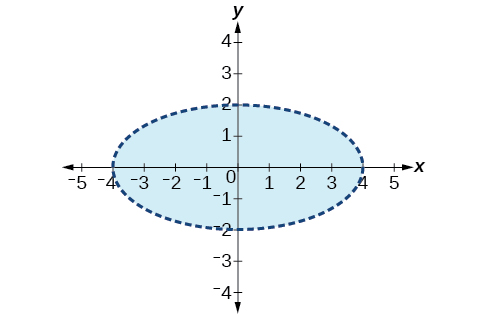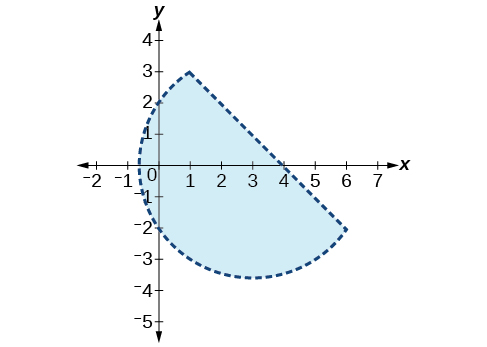# 9.8 Solving systems with cramer's rule  (Page 8/11)

 Page 8 / 11

$\left(10,-10,10\right)$

$\begin{array}{r}\hfill 5x+3y-z=5\text{\hspace{0.17em}}\text{\hspace{0.17em}}\text{\hspace{0.17em}}\\ \hfill 3x-2y+4z=13\\ \hfill 4x+3y+5z=22\end{array}$

$\begin{array}{r}x+y+z=1\\ 2x+2y+2z=1\\ 3x+3y=2\end{array}$

No solutions exist.

$\begin{array}{l}\text{\hspace{0.17em}}\text{\hspace{0.17em}}3x+2y-z=-10\hfill \\ \text{\hspace{0.17em}}\text{\hspace{0.17em}}\text{\hspace{0.17em}}\text{\hspace{0.17em}}x-y+2z=7\hfill \\ -x+3y+z=-2\hfill \end{array}$

$\left(-1,-2,3\right)$

$\begin{array}{r}\hfill 3x+4z=-11\\ \hfill x-2y=5\text{\hspace{0.17em}}\text{\hspace{0.17em}}\text{\hspace{0.17em}}\text{\hspace{0.17em}}\text{\hspace{0.17em}}\text{\hspace{0.17em}}\text{\hspace{0.17em}}\\ \hfill 4y-z=-10\end{array}$

$\begin{array}{r}2x-3y+z=0\\ 2x+4y-3z=0\\ 6x-2y-z=0\end{array}$

$\left(x,\frac{8x}{5},\frac{14x}{5}\right)$

$\begin{array}{r}6x-4y-2z=2\\ 3x+2y-5z=4\\ 6y-7z=5\end{array}$

For the following exercises, write a system of equations to solve each problem. Solve the system of equations.

Three odd numbers sum up to 61. The smaller is one-third the larger and the middle number is 16 less than the larger. What are the three numbers?

11, 17, 33

A local theatre sells out for their show. They sell all 500 tickets for a total purse of $8,070.00. The tickets were priced at$15 for students, $12 for children, and$18 for adults. If the band sold three times as many adult tickets as children’s tickets, how many of each type was sold?

## Systems of Nonlinear Equations and Inequalities: Two Variables

For the following exercises, solve the system of nonlinear equations.

$\begin{array}{l}\begin{array}{l}\\ y={x}^{2}-7\end{array}\hfill \\ y=5x-13\hfill \end{array}$

$\left(2,-3\right),\left(3,2\right)$

$\begin{array}{l}\begin{array}{l}\\ y={x}^{2}-4\end{array}\hfill \\ y=5x+10\hfill \end{array}$

$\begin{array}{l}{x}^{2}+{y}^{2}=16\hfill \\ \text{\hspace{0.17em}}\text{\hspace{0.17em}}\text{\hspace{0.17em}}\text{\hspace{0.17em}}\text{\hspace{0.17em}}\text{\hspace{0.17em}}\text{\hspace{0.17em}}\text{\hspace{0.17em}}\text{\hspace{0.17em}}\text{\hspace{0.17em}}\text{\hspace{0.17em}}\text{\hspace{0.17em}}\text{\hspace{0.17em}}\text{\hspace{0.17em}}\text{\hspace{0.17em}}\text{\hspace{0.17em}}y=x-8\hfill \end{array}$

No solution

$\begin{array}{l}{x}^{2}+{y}^{2}=25\hfill \\ \text{\hspace{0.17em}}\text{\hspace{0.17em}}\text{\hspace{0.17em}}\text{\hspace{0.17em}}\text{\hspace{0.17em}}\text{\hspace{0.17em}}\text{\hspace{0.17em}}\text{\hspace{0.17em}}\text{\hspace{0.17em}}\text{\hspace{0.17em}}\text{\hspace{0.17em}}\text{\hspace{0.17em}}\text{\hspace{0.17em}}\text{\hspace{0.17em}}\text{\hspace{0.17em}}\text{\hspace{0.17em}}y={x}^{2}+5\hfill \end{array}$

$\begin{array}{r}{x}^{2}+{y}^{2}=4\\ y-{x}^{2}=3\end{array}$

No solution

For the following exercises, graph the inequality.

$y>{x}^{2}-1$

$\frac{1}{4}{x}^{2}+{y}^{2}<4$For the following exercises, graph the system of inequalities.

$\begin{array}{l}{x}^{2}+{y}^{2}+2x<3\hfill \\ \text{\hspace{0.17em}}\text{\hspace{0.17em}}\text{\hspace{0.17em}}\text{\hspace{0.17em}}\text{\hspace{0.17em}}\text{\hspace{0.17em}}\text{\hspace{0.17em}}\text{\hspace{0.17em}}\text{\hspace{0.17em}}\text{\hspace{0.17em}}\text{\hspace{0.17em}}\text{\hspace{0.17em}}\text{\hspace{0.17em}}\text{\hspace{0.17em}}\text{\hspace{0.17em}}\text{\hspace{0.17em}}\text{\hspace{0.17em}}\text{\hspace{0.17em}}\text{\hspace{0.17em}}\text{\hspace{0.17em}}\text{\hspace{0.17em}}\text{\hspace{0.17em}}\text{\hspace{0.17em}}\text{\hspace{0.17em}}\text{\hspace{0.17em}}\text{\hspace{0.17em}}\text{\hspace{0.17em}}\text{\hspace{0.17em}}\text{\hspace{0.17em}}y>-{x}^{2}-3\hfill \end{array}$

$\begin{array}{l}{x}^{2}-2x+{y}^{2}-4x<4\hfill \\ \text{\hspace{0.17em}}\text{\hspace{0.17em}}\text{\hspace{0.17em}}\text{\hspace{0.17em}}\text{\hspace{0.17em}}\text{\hspace{0.17em}}\text{\hspace{0.17em}}\text{\hspace{0.17em}}\text{\hspace{0.17em}}\text{\hspace{0.17em}}\text{\hspace{0.17em}}\text{\hspace{0.17em}}\text{\hspace{0.17em}}\text{\hspace{0.17em}}\text{\hspace{0.17em}}\text{\hspace{0.17em}}\text{\hspace{0.17em}}\text{\hspace{0.17em}}\text{\hspace{0.17em}}\text{\hspace{0.17em}}\text{\hspace{0.17em}}\text{\hspace{0.17em}}\text{\hspace{0.17em}}\text{\hspace{0.17em}}\text{\hspace{0.17em}}\text{\hspace{0.17em}}\text{\hspace{0.17em}}\text{\hspace{0.17em}}\text{\hspace{0.17em}}\text{\hspace{0.17em}}\text{\hspace{0.17em}}\text{\hspace{0.17em}}\text{\hspace{0.17em}}\text{\hspace{0.17em}}\text{\hspace{0.17em}}\text{\hspace{0.17em}}\text{\hspace{0.17em}}\text{\hspace{0.17em}}\text{\hspace{0.17em}}\text{\hspace{0.17em}}\text{\hspace{0.17em}}\text{\hspace{0.17em}}y<-x+4\hfill \end{array}$$\begin{array}{l}{x}^{2}+{y}^{2}<1\hfill \\ \text{\hspace{0.17em}}\text{\hspace{0.17em}}\text{\hspace{0.17em}}\text{\hspace{0.17em}}\text{\hspace{0.17em}}\text{\hspace{0.17em}}\text{\hspace{0.17em}}\text{\hspace{0.17em}}\text{\hspace{0.17em}}\text{\hspace{0.17em}}\text{\hspace{0.17em}}\text{\hspace{0.17em}}\text{\hspace{0.17em}}{y}^{2}

## Partial Fractions

For the following exercises, decompose into partial fractions.

$\frac{-2x+6}{{x}^{2}+3x+2}$

$\frac{2}{x+2},\frac{-4}{x+1}$

$\frac{10x+2}{4{x}^{2}+4x+1}$

$\frac{7x+20}{{x}^{2}+10x+25}$

$\frac{7}{x+5},\frac{-15}{{\left(x+5\right)}^{2}}$

$\frac{x-18}{{x}^{2}-12x+36}$

$\frac{-{x}^{2}+36x+70}{{x}^{3}-125}$

$\frac{3}{x-5},\frac{-4x+1}{{x}^{2}+5x+25}$

$\frac{-5{x}^{2}+6x-2}{{x}^{3}+27}$

$\frac{{x}^{3}-4{x}^{2}+3x+11}{{\left({x}^{2}-2\right)}^{2}}$

$\frac{x-4}{\left({x}^{2}-2\right)},\frac{5x+3}{{\left({x}^{2}-2\right)}^{2}}$

$\frac{4{x}^{4}-2{x}^{3}+22{x}^{2}-6x+48}{x{\left({x}^{2}+4\right)}^{2}}$

## Matrices and Matrix Operations

For the following exercises, perform the requested operations on the given matrices.

$A=\left[\begin{array}{rr}\hfill 4& \hfill -2\\ \hfill 1& \hfill 3\end{array}\right],B=\left[\begin{array}{rrr}\hfill 6& \hfill 7& \hfill -3\\ \hfill 11& \hfill -2& \hfill 4\end{array}\right],C=\left[\begin{array}{r}\hfill \begin{array}{cc}6& 7\\ 11& -2\end{array}\\ \hfill \begin{array}{cc}14& 0\end{array}\end{array}\right],D=\left[\begin{array}{rrr}\hfill 1& \hfill -4& \hfill 9\\ \hfill 10& \hfill 5& \hfill -7\\ \hfill 2& \hfill 8& \hfill 5\end{array}\right],E=\left[\begin{array}{rrr}\hfill 7& \hfill -14& \hfill 3\\ \hfill 2& \hfill -1& \hfill 3\\ \hfill 0& \hfill 1& \hfill 9\end{array}\right]$

$-4A$

$\left[\begin{array}{cc}-16& 8\\ -4& -12\end{array}\right]$

$10D-6E$

$B+C$

undefined; dimensions do not match

$AB$

$BA$

undefined; inner dimensions do not match

$BC$

$CB$

$\left[\begin{array}{ccc}113& 28& 10\\ 44& 81& -41\\ 84& 98& -42\end{array}\right]$

$DE$

$ED$

$\left[\begin{array}{ccc}-127& -74& 176\\ -2& 11& 40\\ 28& 77& 38\end{array}\right]$

$EC$

$CE$

undefined; inner dimensions do not match

${A}^{3}$

## Solving Systems with Gaussian Elimination

For the following exercises, write the system of linear equations from the augmented matrix. Indicate whether there will be a unique solution.

$\begin{array}{l}x-3z=7\\ y+2z=-5\text{\hspace{0.17em}}\end{array}$ with infinite solutions

For the following exercises, write the augmented matrix from the system of linear equations.

$\begin{array}{l}\\ \begin{array}{r}\hfill -2x+2y+z=7\\ \hfill 2x-8y+5z=0\\ \hfill 19x-10y+22z=3\end{array}\end{array}$

$\begin{array}{l}\text{\hspace{0.17em}}\text{\hspace{0.17em}}\text{\hspace{0.17em}}\text{\hspace{0.17em}}\text{\hspace{0.17em}}4x+2y-3z=14\hfill \\ -12x+3y+z=100\hfill \\ \text{\hspace{0.17em}}\text{\hspace{0.17em}}\text{\hspace{0.17em}}\text{\hspace{0.17em}}\text{\hspace{0.17em}}9x-6y+2z=31\hfill \end{array}$

$\begin{array}{r}\hfill x+3z=12\text{\hspace{0.17em}}\\ \hfill -x+4y=0\text{\hspace{0.17em}}\text{\hspace{0.17em}}\text{\hspace{0.17em}}\text{\hspace{0.17em}}\\ \hfill y+2z=-7\end{array}$

For the following exercises, solve the system of linear equations using Gaussian elimination.

$\begin{array}{r}3x-4y=-7\\ -6x+8y=14\end{array}$

$\begin{array}{r}3x-4y=1\\ -6x+8y=6\end{array}$

No solutions exist.

$\begin{array}{l}\begin{array}{l}\\ -1.1x-2.3y=6.2\end{array}\hfill \\ -5.2x-4.1y=4.3\hfill \end{array}$

$\begin{array}{r}\hfill 2x+3y+2z=1\text{\hspace{0.17em}}\text{\hspace{0.17em}}\text{\hspace{0.17em}}\text{\hspace{0.17em}}\text{\hspace{0.17em}}\\ \hfill -4x-6y-4z=-2\\ \hfill 10x+15y+10z=0\text{\hspace{0.17em}}\text{\hspace{0.17em}}\text{\hspace{0.17em}}\text{\hspace{0.17em}}\text{\hspace{0.17em}}\end{array}$

No solutions exist.

$\begin{array}{r}\hfill -x+2y-4z=8\text{\hspace{0.17em}}\text{\hspace{0.17em}}\text{\hspace{0.17em}}\text{\hspace{0.17em}}\\ \hfill 3y+8z=-4\\ \hfill -7x+y+2z=1\text{\hspace{0.17em}}\text{\hspace{0.17em}}\text{\hspace{0.17em}}\text{\hspace{0.17em}}\end{array}$

## Solving Systems with Inverses

For the following exercises, find the inverse of the matrix.

$\left[\begin{array}{rr}\hfill -0.2& \hfill 1.4\\ \hfill 1.2& \hfill -0.4\end{array}\right]$

$\frac{1}{8}\left[\begin{array}{cc}2& 7\\ 6& 1\end{array}\right]$

$\left[\begin{array}{rr}\hfill \frac{1}{2}& \hfill -\frac{1}{2}\\ \hfill -\frac{1}{4}& \hfill \frac{3}{4}\end{array}\right]$

$\left[\begin{array}{ccc}12& 9& -6\\ -1& 3& 2\\ -4& -3& 2\end{array}\right]$

No inverse exists.

$\left[\begin{array}{ccc}2& 1& 3\\ 1& 2& 3\\ 3& 2& 1\end{array}\right]$

what is set?
a colony of bacteria is growing exponentially doubling in size every 100 minutes. how much minutes will it take for the colony of bacteria to triple in size
I got 300 minutes. is it right?
Patience
no. should be about 150 minutes.
Jason
It should be 158.5 minutes.
Mr
ok, thanks
Patience
100•3=300 300=50•2^x 6=2^x x=log_2(6) =2.5849625 so, 300=50•2^2.5849625 and, so, the # of bacteria will double every (100•2.5849625) = 258.49625 minutes
Thomas
what is the importance knowing the graph of circular functions?
can get some help basic precalculus
What do you need help with?
Andrew
how to convert general to standard form with not perfect trinomial
can get some help inverse function
ismail
Rectangle coordinate
how to find for x
it depends on the equation
Robert
yeah, it does. why do we attempt to gain all of them one side or the other?
Melissa
whats a domain
The domain of a function is the set of all input on which the function is defined. For example all real numbers are the Domain of any Polynomial function.
Spiro
Spiro; thanks for putting it out there like that, 😁
Melissa
foci (–7,–17) and (–7,17), the absolute value of the differenceof the distances of any point from the foci is 24.
difference between calculus and pre calculus?
give me an example of a problem so that I can practice answering
x³+y³+z³=42
Robert
dont forget the cube in each variable ;)
Robert
of she solves that, well ... then she has a lot of computational force under her command ....
Walter
what is a function?
I want to learn about the law of exponent
explain this
what is functions?
A mathematical relation such that every input has only one out.
Spiro
yes..it is a relationo of orders pairs of sets one or more input that leads to a exactly one output.
Mubita
Is a rule that assigns to each element X in a set A exactly one element, called F(x), in a set B.
RichieRich

#### Get Jobilize Job Search Mobile App in your pocket Now!ByByBy Christine ZeelieBy Saylor FoundationBy Ann SchlosserByBy Janet ForresterBy Donyea SweetsBy Mary MateraBy OpenStaxBy Richley CrapoBy Eric Crawford